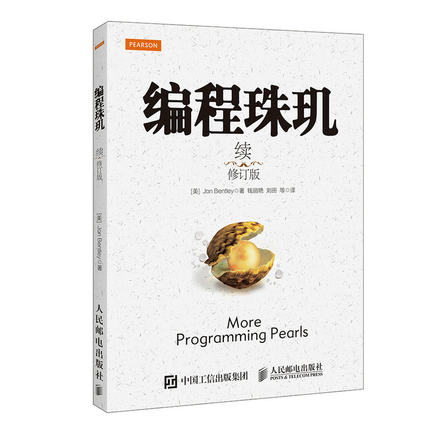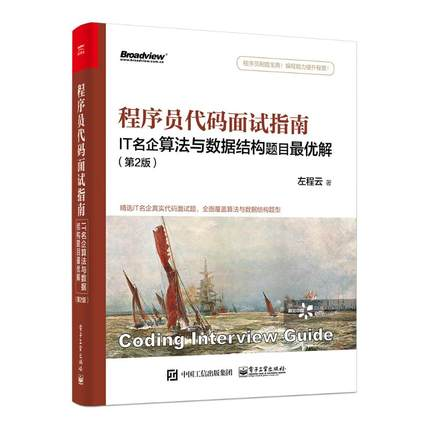# 大学四年，小白一路走来，分享一波我是如何学习、提高自己的算法

### 切勿盲目刷题：刷题前的知识积累

1、常见数据结构：链表、树(如二叉树)。（是的，链表和二叉树是重点，图这些可以先放着）

2、常见算法思想：贪婪法、分治法、穷举法、动态规划，回溯法。（贪婪、穷举、分治是基础，动态规划有难度，可以先放着）

1、数据结构与算法分析（c 语言描述版）2、挑战程序设计竞赛3、编程之美4、编程珠玑5、程序员代码面试指南：IT 名企算法与数据结构题目最优解### AC不是目的，我们要追求完美

public static int solve(int n){
if(n == 1 || n == 2){
return n;
}else if(n <= 0){
return 0;
}else{
return solve(n-1) + solve(n-2);
}
}


//用一个HashMap来保存已经计算过的状态
static Map<Integer,Integer> map = new HashMap();
public static int solve(int n){
if(n <= 0)return 0;
else if(n <= 2){
return n;
}else{//是否计算过
if(map.containsKey(n)){
return map.get(n);
}else{
int m = solve(n-1) + solve(n-2);
map.put(n, m);
return m;
}
}
}



public static int solve(int n){
if(n <= 0)
return 0;
if(n <= 2){
return n;
}

int f1 = 0;
int f2 = 1;
int sum = 0;
for(int i = 1; i<= n; i++){
sum = f1 + f2;
f1 = f2;
f2 = sum;
}
return sum;
}


1、在刷题的时候，我们要力求完美。

2、我想不到这些方法啊，怎么办？那么你就可以去看别人的做法，之后，遇到类似的题，你就会更有思路，更知道往哪个方向想。

3、可以从简单暴力入手做一道题，在考虑空间与时间之间的衡量，一点点去优化。

leetcode有中文版

### 学习一些解题技巧

#### 1、找出没有重复的数

123451234 = （11)(22)(33)(44)5= 00005 = 5。

int find(int[] arr){
int tmp = arr;
for(int i = 1;i < arr.length; i++){
tmp = tmp ^ arr[i];
}
return tmp;
}


#### 2、m的n次方

int pow(int n){
int tmp = 1;
for(int i = 1; i <= n; i++) {
tmp = tmp * m;
}
return tmp;
}


m^1101 = m^0001 * m^0100 * m^1000。

int pow(int n){
int sum = 1;
int tmp = m;
while(n != 0){
if(n & 1 == 1){
sum *= tmp;
}
tmp *= tmp;
n = n >> 1;
}

return sum;
}


【算法技巧】位运算装逼指南

### 再说数据结构发重要性

1、链表（如单向链表、双向链表）。

2、树（如二叉树、平衡树、红黑树）。

3、图（如最短路径的几种算法）。

4、队列、栈、矩阵。

### 看完有收获？那么希望老铁别吝啬你的三连击哦

1、点赞，可以让更多的人看到这篇文章
2、关注我的原创微信公众号『苦逼的码农』，第一时间阅读我的文章。公众号后台回复『电子书』，还送你一份电子书大礼包哦。
3、也欢迎关注我的博客哦。### 作者简洁12-18
02-06709
01-061946
12-181万+
12-063463
06-041203
12-2617万+
07-132万+
06-161万+
02-2388
06-10910
09-113万+
02-201万+
01-3016万+
11-201万+
12-014270
07-016416
©️2020 CSDN 皮肤主题: Age of Ai 设计师:meimeiellie